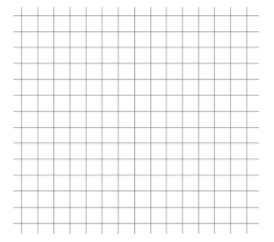# Equations and Inequalities

## Objective

Write equations for and graph ratio situations. Define independent and dependent variables.

## Common Core Standards

### Core Standards

?

• 6.EE.C.9 — Use variables to represent two quantities in a real-world problem that change in relationship to one another; write an equation to express one quantity, thought of as the dependent variable, in terms of the other quantity, thought of as the independent variable. Analyze the relationship between the dependent and independent variables using graphs and tables, and relate these to the equation. For example, in a problem involving motion at constant speed, list and graph ordered pairs of distances and times, and write the equation d = 65t to represent the relationship between distance and time.

• 6.RP.A.3.A — Make tables of equivalent ratios relating quantities with whole number measurements, find missing values in the tables, and plot the pairs of values on the coordinate plane. Use tables to compare ratios.

?

• 5.OA.B.3

• 6.RP.A.3

• 6.NS.C.6.C

## Criteria for Success

?

1. Use equivalent ratios to complete ratio tables.
2. Plot values from a table on a coordinate plane.
3. Write equations to represent the relationship between two associated quantities.
4. Define and identify the independent variable in a relationship.
5. Define and identify the dependent variable in a relationship.

## Tips for Teachers

?

This lesson brings together several concepts and skills that students have worked on throughout the year, such as tables of equivalent values (6.RP.3), writing equations (6.EE.1), plotting points (6.NS.8), and determining values in ratio relationships (6.RP.3). The novelty is not in any one specific concept or skill but rather in how they interact and represent one another in new ways. For example, after studying equations, students can now use an equation to represent how two quantities in a ratio situation are related to one another, they can show this visually in the coordinate plane, and analyze the relationship to draw conclusions (MP.4).

#### Fishtank Plus

• Problem Set
• Student Handout Editor
• Vocabulary Package

## Anchor Problems

?

### Problem 1

A recipe for sugar cookies calls for 1 cup of sugar for every 2 cups of flour.

 Sugar (cups) 1 8 Flour (cups) 6 10 20

1. Use the ratio to complete the table.
2. If you know the number of cups of sugar, $s$, in the recipe, how can you determine the number of cups of flour, $f$, to use? What equation represents this relationship?
3. If you know the number of cups of flour, $f$, in the recipe, how can you determine the number of cups of sugar, $s$, to use? What equation represents this relationship?
4. In each equation, what is the independent variable and what is the dependent variable?

### Problem 2

Julianna participated in a walk-a-thon to raise money for cancer research. She recorded the total distance she walked at several different points in time, but a few of the entries got smudged and can no longer be read. The times and distances that can still be read are listed in the table below.

 Time (hours) Miles Walked 1 2 6 12 5
1. Assume Julianna walked at a constant speed. Complete the table and plot Julianna’s progress in the coordinate plane.1. How fast was Julianna walking in miles per hour? How long did it take Julianna to walk 1 mile?
2. Next year Julianna is planning to walk for 7 hours. If she walks at the same speed next year, how many miles will she walk?

#### References

Illustrative Mathematics Walk-A-Thon 1

Walk-A-Thon 1, accessed on Feb. 28, 2018, 4:21 p.m., is licensed by Illustrative Mathematics under either the CC BY 4.0 or CC BY-NC-SA 4.0. For further information, contact Illustrative Mathematics.

## Problem Set

?The following resources include problems and activities aligned to the objective of the lesson that can be used to create your own problem set.

?

To make green paint, a paint store combines blue and yellow paint in a specific ratio, as shown in the table below.

 Blue Paint (pints) Yellow Paint (pints) 2 3 4 6 6 9 8 12
1. If the store uses 21 pints of yellow paint, how many pints of blue paint should they use?
2. If the store uses 20 pints of blue paint, how many pints of yellow paint should they use?
3. Write an equation to represent the relationship between blue and yellow paint. Identify which variable is your independent variable and which is your dependent variable.
4. Plot the values from the table in the coordinate plane. Label the axes with your independent and dependent variables.?